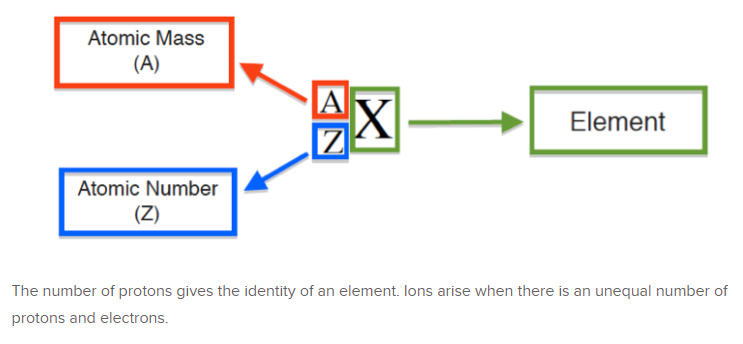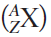# Problem: Write the atomic symbol  for each of the isotopes described below.a. number of protons = 27, number of neutrons = 31

###### FREE Expert Solution
• Recall that in nuclear notation:• Atomic mass can be calculated by getting the sum of # of protons and neutrons.
• Atomic # can give us the identity of the element where it is also equal to the # of protons (atomic # = # of protons)
86% (304 ratings)###### Problem Details

Write the atomic symbolfor each of the isotopes described below.

a. number of protons = 27, number of neutrons = 31